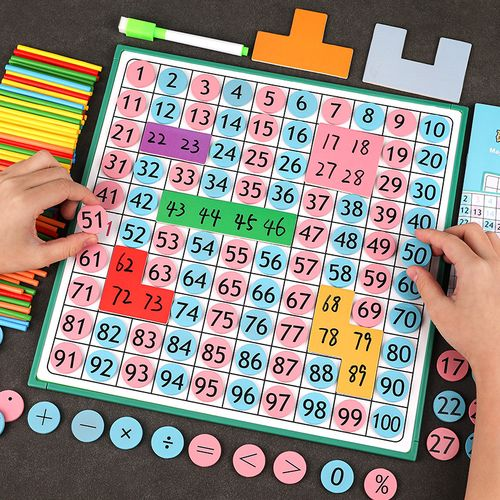# Guess My Number

By Math Is Fun, May 14, 2022

I wrote down a number on a piece of paper, “It’s a number between 1 and 100.” I asked my son, “Your job is to guess the number. Each time you make a guess, I can tell you whether it’s correct, or if your guess is higher or lower than my number. How many times do you need to guess before finding out what the number is?”

“I can ask, is it the number 1, is it the number 2..is it the number 100? I can of course get the number since it’s between 1 and 100.” Son started thinking.

“OK, if my number is 100, you need to ask 99 times. Can you do better?”

“I can ask: is it 10? If you say my guess is too high, I know it’s between 1 and 9.”

“Excellent! What if it’s 99? Then I will say your guess is too low, and you still have to guess between 11 and 100.”

“Right, so I should ask: is it 50? Whether the guess is high or low, I can always decrease the possible numbers.”

“Cool! You cut  the range in half.” I agreed. “Your guess is low. What would be your next question?”

“Is it 75?”

“Cool, I think you’ve got the idea! Always take the midpoint of the remaining range.”

Here is the summary of my son’s guesses and my answers:

Son was particularly interested in this game. He asked me to play this game again and again. After half a dozen games, I asked him, “So how many guesses do you need for any number between 1 and 100?”

“Sometimes I got lucky, like the time you had the number 50 on the paper. I got it in one guess. But sometimes it took several questions, I think 7?”

“Right, I can tell you you can always find the right number in 7 guesses. This is called a binary search. You always pick the midpoint of the range as your guess. No matter what the number is, you can always cut the range in half for the next guess.”

Guessing a number between 1 and 100 is a problem. To solve a problem, you can take different approaches. Each approach is called an algorithm. We tried two algorithms today

• We can guess 1, 2, 3 and all the way to 99. This might take up to 99 guesses if the number is 100.
• We can use the binary search algorithm we just described. In that case, 7 guesses is the maximum number needed for any number.

Obviously the second algorithm is faster and better. Finding the best algorithm is an important subject for mathematics and computer science.